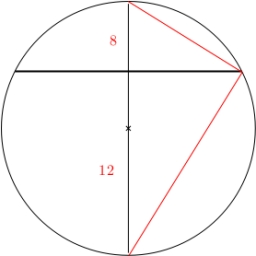# Solutions 45511

Two parallel chords in a circle with a radius of 6 cm have lengths of 6 cm and 10 cm. Calculate their distance from each other. Find both solutions.

x =  8.5128 cm
y =  1.8795 cm

### Step-by-step explanation:Did you find an error or inaccuracy? Feel free to write us. Thank you!

Tips for related online calculators
Do you want to convert length units?
The Pythagorean theorem is the base for the right triangle calculator.

#### Grade of the word problem:

We encourage you to watch this tutorial video on this math problem: